## How to Calculate and Solve for Water Convergence efficiency | Methods of Application of Water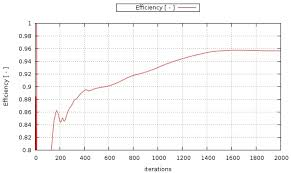The image above represents water convergence efficiency.

To compute for water convergence efficiency, two essential parameters are needed and these parameters are Water delivered to the Irrigated Plot (wfand Water delivered from the Source (wd).

The formula for calculating water convergence efficiency:

Ec = 100 . wf / wd

Where:

Ec = Water Convergence Efficiency
wf = Water delivered to the Irrigated Plot
wd = Water delivered from the Source

Let’s solve an example;
Find the water convergence efficiency when the water delivered to the irrigated plot is 30 and the water delievered from the source is 10.

This implies that;

wf = Water delivered to the Irrigated Plot = 30
wd = Water delivered from the Source = 10

Ec = 100 . wf / wd
Ec = 100 . (30) / 10
Ec = 3000 / 10
Ec = 300

Therefore, the water convergence efficiency is 300%.

Calculating the Water Delivered to the Irrigated Plot when the Water Convergence Efficiency and the Water Delivered from the Source is Given.

wf = Ec x wd / 100

Where;

wf = Water delivered to the Irrigated Plot
Ec = Water Convergence Efficiency
wd = Water delivered from the Source

Let’s solve an example;
Find the water delivered to the irrigated plot when the water convergence efficiency is 20 and the water delivered from the source is 5.

This implies that;

Ec = Water Convergence Efficiency = 20
wd = Water delivered from the Source = 5

wf = Ec x wd / 100
wf = 20 x 5 / 100
wf = 100 / 100
wf = 1

Therefore, the water delivered to the irrigated plot is 1%.

## How to Calculate and Solve for Water Application Efficiency | Methods of Application of Water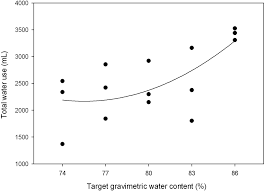The image above represents water application efficiency.

To compute for water application efficiency, two essential parameters are needed and these parameters are Water stored in the Root Zone of the Plant (wsand Water delivered to the Irrigated Plot (wf).

The formula for calculating water application efficiency:

Ea = ws / wf

Where:

Ea = Water Application Efficiency
ws = Water stored in the Root Zone of the Plant
wf = Water delivered to the Irrigated Plot

Let’s solve an example;
Find the water application efficiency when the water stored in the root zone of the plant is 45 and the water delivered to the irrigated plot is 9.

This implies that;

ws = Water stored in the Root Zone of the Plant = 45
wf = Water delivered to the Irrigated Plot = 9

Ea = ws / wf
Ea = 45 / 9
Ea = 5

Therefore, the water application efficiency is 5.

Calculating the Water Stored in the Root Zone of the Plant when the Water Application Efficiency and the Water Delivered to the Irrigated Plot is Given.

ws = Ea x wf

Where;

ws = Water stored in the Root Zone of the Plant
Ea = Water Application Efficiency
wf = Water delivered to the Irrigated Plot

Let’s solve an example;
Find the water stored in the root zone of the plant when the water application efficiency is 15 and the water delivered to the irrigated plot is 4.

This implies that;

Ea = Water Application Efficiency = 15
wf = Water delivered to the Irrigated Plot = 4

ws = Ea x wf
ws = 15 x 4
ws = 60

Therefore, the water stored in the root zone of the plant is 60.

## How to Calculate and Solve for Evapotranspiration | Jensen Haide Method | Methods of Application of Water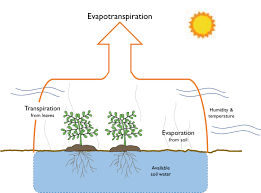The image above represents evapotranspiration.

To compute for evapotranspiration, four essential parameters are needed and these parameters are Temperature co-efficient (ct), Mean dialy temperature (T), Intercept on the Temperature (Txand Incident solar radiation (Rs).

The formula for calculating evapotranspiration:

Etp = ct(T – Tx)Rs

Where:

Etp = Evapotranspiration
ct = Temperature Co-efficient
T = Mean Daily Temperature
Tx = Intercept on the Temperature

Let’s solve an example;
Find the evapotranspiration when the temperature co-efficient is 11, the mean dialy temperature is 21, the intercept on the temperature is 7 and the incident solar radiation is 4.

This implies that;

ct = Temperature Co-efficient = 11
T = Mean Daily Temperature = 21
Tx = Intercept on the Temperature = 7
Rs = Incident Solar Radiation = 4

Etp = ct(T – Tx)Rs
Etp = 11(21 – 7)(4)
Etp = 11(14)(4)
Etp = 616

Therefore, the evapotranspiration is 616.

Calculating the Temperature Co-efficient when the Evapotranspiration, the Mean Dialy Temperature, the Intercept on the Temperature and the Incident Solar Radiation is Given.

ct = Etp / (T – Tx) Rs

Where;

ct = Temperature Co-efficient
Etp = Evapotranspiration
T = Mean Daily Temperature
Tx = Intercept on the Temperature

Let’s solve an example;
Find the temperature co-efficient when the evapotranspiration is 44, the mean daily temperature is 20, the intercept on the temperature is 12 and the incident solar radiation is 4.

This implies that;

Etp = Evapotranspiration = 44
T = Mean Daily Temperature = 20
Tx = Intercept on the Temperature = 12
Rs = Incident Solar Radiation = 4

ct = Etp / (T – Tx) Rs
ct = 44 / (20 – 12) 4
ct = 44 / (8) 4
ct = 44 / 32
ct = 1.375

Therefore, the temperature co-efficient is 1.375.

## How to Calculate and Solve for Weighing Factor | Methods of Application of Water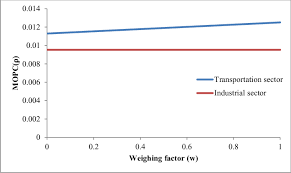The image above represents weighing factor.

To compute for weighing factor, two essential parameters are needed and these parameters are Slope of Saturated Vapour Pressure versus Temperature Curve (D) and Psychometric Constant (Y).

The formula for calculating weighing factor:

W = D / (D + Y)

Where:

W = Weighing Factor
D = Slope of Saturated Vapour Pressure versus Temperature Curve
Y = Psychometric Constant

Let’s solve an example;
Find the weighing factor when the slope of saturated vapour pressure versus temperature curve is 14 and the psychometric constant is 18.

This implies that;

D = Slope of Saturated Vapour Pressure versus Temperature Curve = 14
Y = Psychometric Constant = 18

W = D / (D + Y)
W = 14 / (14 + 18)
W = 14 / 32
W = 0.4375

Therefore, the weighing factor is 0.4375.

## How to Calculate and Solve for Evapotranspiration | Modified Penman Method | Methods of Application of Water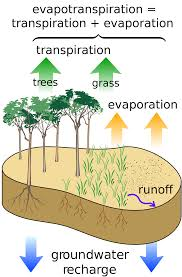The image above represents evapotranspiration.

To compute for evapotranspiration, five essential parameters are needed and these parameters are Weighing Factor (W), Net Radiation (Rn), Function Value of Wind Velocity (f(u)), Saturated Vapour Pressure at Mean Density Pressure (ρsand Actual Vapour Pressure of the Air (ρa).

The formula for calculating evapotranspiration:

Etp = WRn + (1 – W)f(u)(ρs – ρa)

Where:

Etp = Evapotranspiration
W = Weighing Factor
f(u) = Function Value of Wind Velocity
ρs = Saturated Vapour Pressure at Mean Density Pressure
ρa = Actual Vapour Pressure of the Air

Let’s solve an example;
Find the evapotranspiration when the weighing factor is 7, the net radiation is 13, the function value of wind velocity is 11, saturated vapour pressure at mean density pressure is 8 and the actual vapour pressure of the air is 21.

This implies that;

W = Weighing Factor = 7
Rn = Net Radiation = 13
f(u) = Function Value of Wind Velocity = 11
ρs = Saturated Vapour Pressure at Mean Density Pressure = 8
ρa = Actual Vapour Pressure of the Air = 21

Etp = WRn + (1 – W)f(u)(ρs – ρa)
Etp = 7(13) + (1 – 7)(11)(8 – 21)
Etp = 91 + (-6)(11)(-13)
Etp = 91 + 858
Etp = 949

Therefore, the evapotranspiration is 949.

## How to Calculate and Solve for Frequency of Irrigation | Methods of Application of Water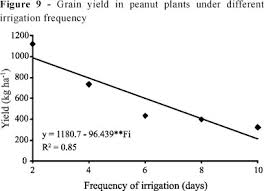The image above represents frequency of irrigation.

To compute for frequency of irrigation, two essential parameters are needed and these parameters are Minimum Depth of Water (dwand Depth of Water for Specified Period (cu).

The formula for calculating frequency of irrigation:

fw = dw / cu

Where:

fw = Frequency of Irrigation
dw = Minimum Depth of Water
cu = Depth of Water for Specified Period

Let’s solve an example;
Find the frequency of irrigation when the minimum depth of water is 22 and the depth of water for specified period is 44.

This implies that;

dw = Minimum Depth of Water = 22
cu = Depth of Water for Specified Period = 44

fw = dw / cu
fw = 22 / 44
fw = 0.5

Therefore, the frequency of irrigation is 0.5.

Calculating for the Minimum Depth of Water when the Frequency of Irrigation and the Depth of Water for Specified Period is Given.

dw = fw x cu

Where;

dw = Minimum Depth of Water
fw = Frequency of Irrigation
cu = Depth of Water for Specified Period

Let’s solve an example;
Find the minimum depth of water when the frequency of irrigation is 12 and the depth of water for specified period is 9.

This implies that;

fw = Frequency of Irrigation = 12
cu = Depth of Water for Specified Period = 9

dw = fw x cu
dw = 12 x 9
dw = 108

Therefore, the minimum depth of water is 108.

## How to Calculate and Solve for Minimum Depth of Water | Methods of Application of Water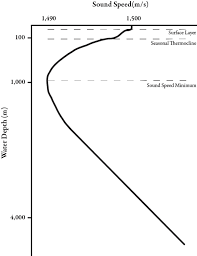The image above represents minimum depth of water.

To compute for minimum depth of water, three essential parameters are needed and these parameters are Specific Weight of Soil (ws), Specific Weight of Water (w) and Depth of Root Zone (d).

The formula for calculating minimum depth of water:

dw = ws . d / w

Where:

dw = Minimum Depth of Water
ws = Specific Weight of Soil
w = Specific Weight of Water
d = Depth of Root Zone

Let’s solve an example;
Find the minimum depth of water when the specific weight of soil is 12, the specific weight of water is 14 and the depth of root zone is 16.

This implies that;

ws = Specific Weight of Soil = 12
w = Specific Weight of Water = 14
d = Depth of Root Zone = 16

dw = ws . d / w
dw = 12 (16) / 14
dw = 192 / 14
dw = 13.71

Therefore, the minimum depth of water is 13.71.

Calculating the Specific Weight of Soil when the Minimum Depth of Water, the Specific Weight of Water and the Depth of Root Zone is Given.

ws = dw x w / d

Where;

ws = Specific Weight of Soil
dw = Minimum Depth of Water
w = Specific Weight of Water
d = Depth of Root Zone

Let’s solve an example;
Find the specific weight of soil when the minimum depth of water is 24, the specific weight of water is 6 and the depth of root zone is 12.

This implies that;

dw = Minimum Depth of Water = 24
w = Specific Weight of Water = 6
d = Depth of Root Zone = 12

ws = dw x w / d
ws = 24 x 6 / 12
ws = 144 / 12
ws = 12

Therefore, the specific weight of soil is 12.

## How to Calculate and Solve for Green Ampt Infiltration Model | Methods of Application of Water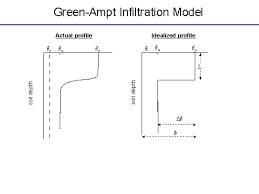The image above represents green ampt infiltration model.

To compute for green ampt infiltration model, three essential parameters are needed and these parameters are Value (Ks), Distance from the Ground Surface to the Wetting Front (L) and Capillary Suction at the Wetting Front (S).

The formula for calculating green ampt infiltration model:

Fp = Ks(L + S) / L

Where:

Fp = Green Ampt Infiltration Model
Ks = Value
L = Distance from the Ground Surface to the Wetting Front
S = Capillary Suction at the Wetting Front

Let’s solve an example;
Find the green ampt infiltration model when the value is 21, the distance from the ground surface to the wetting front is 15 and capillary suction at the wetting front is 4.

This implies that;

Ks = Value = 21
L = Distance from the Ground Surface to the Wetting Front = 15
S = Capillary Suction at the Wetting Front = 4

Fp = Ks(L + S) / L
Fp = 21(15 + 4) / 15
Fp = 21(19) / 15
Fp = 399 / 15
Fp = 26.6

Therefore, the green ampt infiltration model is 26.6.

## How to Calculate and Solve for Infiltration Capacity | Methods of Application of Water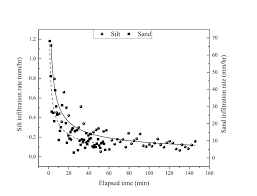The image above represents infiltration capacity.

To compute for infiltration capacity, four essential parameters are needed and these parameters are Initial infiltration capacity (Fo), Final constant infiltration capacity (Fc), Time (t) and Empirical constant (k).

The formula for calculating infiltration capacity:

Fp = Fc + (Fo – Fc)-kt

Where:

Fp = Infiltration Capacity
Fo = Initial Infiltration Capacity
Fc = Final Constant Infiltration Capacity
t = Time
k = Empirical Constant

Let’s solve an example;
Find the infiltration capacity when the initial infiltration capacity is 16, the final constant infiltration capacity is 10, the time is 6 and the empirical constant is 21.

This implies that;

Fo = Initial Infiltration Capacity = 16
Fc = Final Constant Infiltration Capacity = 10
t = Time = 6
k = Empirical Constant = 21

Fp = Fc + (Fo – Fc)-kt
Fp = 10 + (16 – 10)-(21)(6)
Fp = 10 + (6)-126
Fp = 10 + 8.97e-99
Fp = 10

Therefore, the infiltration capacity is 10.

## How to Calculate and Solve for Weir Discharge | Methods of Application of Water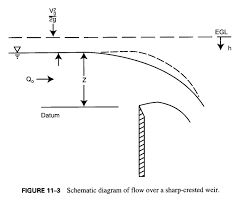The image above represents weir discharge.

To compute for weir discharge, three essential parameters are needed and these parameters are Co-efficient of weir (C), Length of the weir (L) and Head of water over the crest (H).

The formula for calculating weir discharge:

Q = CLH1.5

Where:

Q = Weir Discharge
C = Co-efficient of Weir
L = Length of the Weir
H = Head of Water over the Crest

Let’s solve an example;
Find the weir discharge when the co-efficient of weir is 14, the lenght of the weir is 22 and the head of water over the crest is 8.

This implies that;

C = Co-efficient of Weir = 14
L = Length of the Weir = 22
H = Head of Water over the Crest = 8

Q = CLH1.5
Q = (14)(22)(8)1.5
Q = (14)(22)(22.62)
Q = 6969.2

Therefore, the weir discharge is 6969.2.

Calculating for the Co-efficient of Weir when the Weir Discharge, the Length of the Weir and the Head of Water over the Crest is Given.

C = Q / LH1.5

Where;

C = Co-efficient of Weir
Q = Weir Discharge
L = Length of the Weir
H = Head of Water over the Crest

Let’s solve an example;
Find the co-efficient of weir when the weir discharge is 40, the length of the weir is 14 and the head of water over the crest is 8.

This implies that;

Q = Weir Discharge = 40
L = Length of the Weir = 14
H = Head of Water over the Crest = 8

C = Q / LH1.5
C = 40 / 14 x 81.5
C = 40 / 14 x 22.62
C = 40 / 316.68
C = 0.126

Therefore, the co-efficient of weir is 0.126.